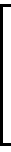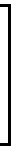# Linear Equations System Solver

 This script allow you to solve an n x n equation system ([A]À[X]=[B]) by means of inversion of the coefficient matrix A The system must be writing of the [A]À[X]=[B] form In order to solve the system you must introduce the coefficients of each matrix. Write the coefficient separately by means of [space] or [enter] key. (see the example)Put here the coefficient ... a11[spc] a12[spc] ...[enter] a21[spc]Àx[enter] y[enter] z ...=Put here the results of the system[A] À [X] = [B]
 [X] = [A]-1 À [B]=À=[X] = [A]-1À[B]